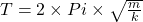## A mass m on a spring with a spring constant k is in simple harmonic motion with a period T. If the same mass is hung from a spring with a sp

Question

A mass m on a spring with a spring constant k is in simple harmonic motion with a period T. If the same mass is hung from a spring with a spring constant of 3k, the period of the motion will be _______.

in progress 0
5 months 2021-08-26T10:34:12+00:00 1 Answers 0 views 0

d) decreased by a factor of the square root of 3

Explanation:

Data provided in the question

Mass = M on a spring

Spring constant = k

Period = T

Spring constant = 3k in the case when the same mass is hung

Based on the above information, the period of the motion is

As we know thatBased on this, there is a decrease by a factor of the square root of 3

hence, the correct option is d.